# Texas Go Math Grade 1 Lesson 6.4 Answer Key Doubles Plus 1 and Doubles Minus 1

Refer to our Texas Go Math Grade 1 Answer Key Pdf to score good marks in the exams. Test yourself by practicing the problems from Texas Go Math Grade 1 Lesson 6.4 Answer Key Doubles Plus 1 and Doubles Minus 1.

## Texas Go Math Grade 1 Lesson 6.4 Answer Key Doubles Plus 1 and Doubles Minus 1

Essential Question
How can you use the doubles fact, 4 + 4, to solve each problem? Draw to show how Complete the addition sentence.
Explanation:
4 + 4 = 8
4 + 4  is a double fact
a number is added to itself is a double fact

Explore
How can you use the doubles fact, 4 + 4, to solve each problem? Draw to show how. Complete the addition sentence.Explanation:
4 + 9
this is the example of double plus one
4 + 3 = 7
this is the example of double minus one

For The Teacher

• Read the problems. Look at the doubles fact. 4 + 4. Draw to show 1 more in the first workspace. Draw to show 1 less in the second workspace.

Math Talk
Mathematical Processes
Explain what happens to the doubles fact when you increase one addend by one or decrease one addend by one.
Explanation:
4 + 9
this is the example of double plus one
4 + 3 = 7
this is the example of double minus one

Model and Draw

Use the doubles fact 5 + 5 to add.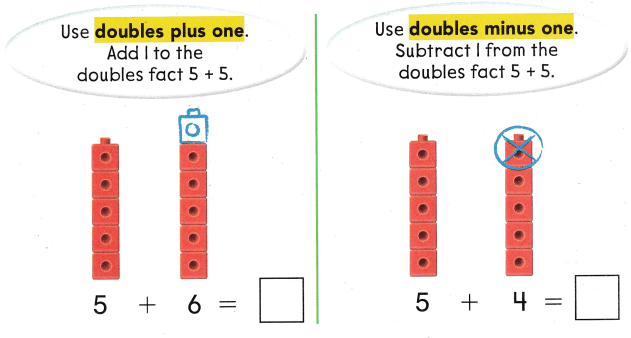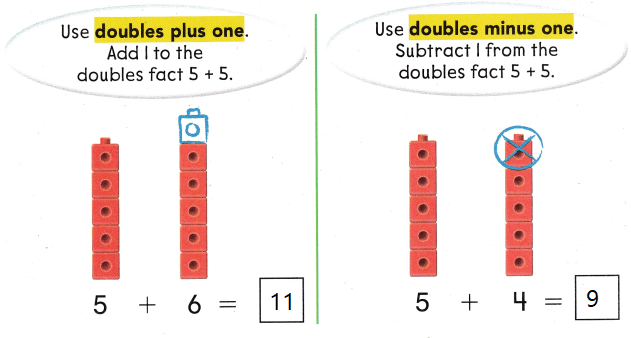Explanation:
5 + 6 = 11
double plus one 5 + 5 + 1 = 11
5 + 4 = 9
double minus one 5 + 5 – 1 = 9

Share and Show

Useto add. Solve the doubles fact.
Then use doubles plus one or doubles minus one. Circle + or — to show how you solved each one.

Question 1.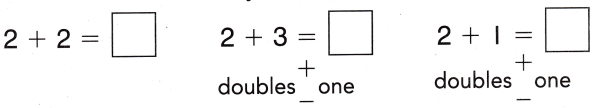Explanation:
use doubles plus one or doubles minus one
circle the appropriate symbol
2 + 2 = 4
2 + 2 + 1 = 5
2 + 2 – 1 = 3

Question 2.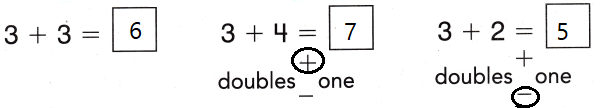Explanation:
use doubles plus one or doubles minus one
circle the appropriate symbol
3 + 3 = 6
3 + 3 + 1 = 7
3 + 3 – 1 = 5

Question 3.Explanation:
use doubles plus one or doubles minus one
circle the appropriate symbol
4 + 4 = 8
4 + 4 + 1 = 9
4 + 4 – 1 = 7

Problem Solving

Add. Write the doubles fact you used to solve the problem.

Question 4.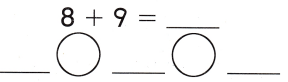Explanation:
The number added to itself is called double fact
8 + 9 = 17
8 + 8 + 1 = 17

Question 5.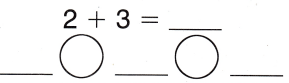Explanation:
The number added to itself is called double fact
2 + 3 = 5
2 + 2 + 1 = 5

Question 6.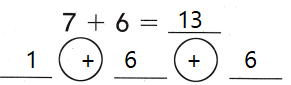Explanation:
The number added to itself is called double fact
7 + 6 = 13
6 + 6 + 1 = 13

Question 7.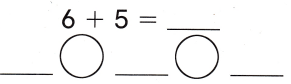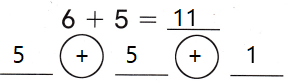Explanation:
The number added to itself is called double fact
6 + 5 = 11
5 + 5 + 1 = 11

Question 8.Explanation:
The number added to itself is called double fact
3 + 4 = 7
3 + 3 + 1 = 7

Question 9.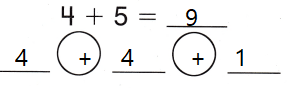Explanation:
The number added to itself is called double fact
4 + 5 = 9
4 + 4 + 1 = 9

Write the addition sentence to solve.

Question 10.
H.O.T. Brianna has 6 toy ducks. Ian has the same number of toy ducks and a toy fish. How many toys do Brianna and Ian have?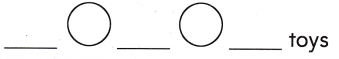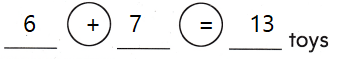Explanation:
Brianna has 6 toy ducks. Ian has the same number of toy ducks
6 + 6 = 12
and a toy fish.
12 + 1 = 13
6 + 6 + 1
6 + 7 = 13
13 toys that Brianna and Ian have

Question 11.
H.O.T. Multi-Step Add. Write the doubles plus one fact. Write the doubles minus one fact.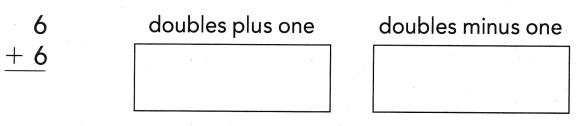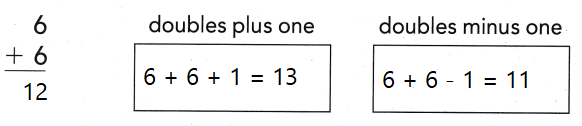Explanation:
use doubles plus one or doubles minus one
6 + 6 = 12
6 + 6 + 1 = 13
6 + 6 – 1 = 11

Question 12.
Alexis wins 3 games of checkers. Miranda wins 4 games. How many games did they play?
(A) 5
(B) 6
(C) 7
Explanation:
Alexis wins 3 games of checkers. Miranda wins 4 games.
3 + 3 + 1 = 7
7 games they play

Question 13.
Analyze Which doubles fact helps you solve the problem?
6 ducks swim on a pond. 7 more ducks join them. How many ducks are there now?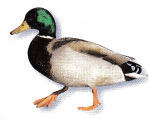(A) 5 + 5 = 10
(B) 6 + 6 = 12
(C) 8 + 8 = 16
Explanation:
6 + 6 = 12
6 + 6 + 1 = 13
13 ducks are there now

Question 14.
Which doubles fact helps you solve the problem?
How many books do they buy?(A) 2 + 2 = 4
(B) 3 + 3 = 6
(C) 5 + 5 = 10
Explanation:
4 + 4 + 1 = 9
5 + 5 – 1  = 9
10 – 1 = 9

Question 15.
Texas Test Prep Which doubles fact helps you solve 6 + 7 = 13?
(A) 5 + 5 = 10
(B) 6 + 6 = 12
(C) 8 + 8 = 16
Explanation:
6 + 6 + 1 = 13
12 + 1 = 13
6 + 7 = 13

Take Home Activity

• Have your child explain how to use a doubles fact to solve the doubles plus one fact 4 + 5 and the doubles minus one fact 4 + 3.
Explanation:
4 + 4 = 8
4 + 4 + 1 = 9
4 + 4 – 1 = 7

### Texas Go Math Grade 1 Lesson 6.4 Homework and Practice Answer Key

Solve the doubles fact. Then use doubles plus one or doubles minus one. Circle + or – to show how you solved each one.

Question 1.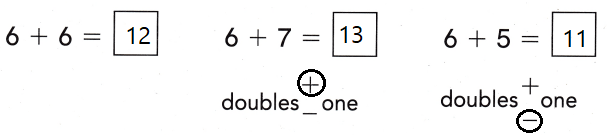Explanation:
use doubles plus one or doubles minus one
circle the appropriate symbol
6 + 6 = 12
6 + 6 + 1 = 13
6 + 6 – 1 = 11

Question 2.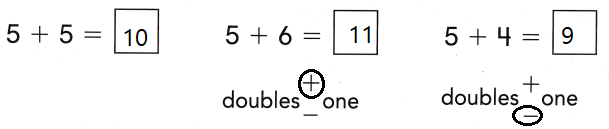Explanation:
use doubles plus one or doubles minus one
circle the appropriate symbol
5 + 5 = 10
5 + 5 + 1 = 11
5 + 5 – 1 = 9

Problem Solving

Write the addition sentence to solve.

Question 3.
Mrs. Jones has 9 cans of soup. She has 8 cans of fruit. How many cans does Mrs. Jones have?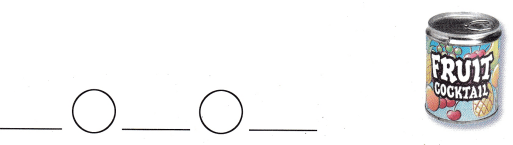Explanation:
Mrs. Jones has 9 cans of soup. She has 8 cans of fruit.
8 + 8 + 1 = 17
16 + 1 = 17
17  cans that Mrs. Jones have

Texas Test Prep

Lesson Check

Question 4.
There are 5 soccer balls. There are 4 basketballs. How many balls are there?
(A) 8
(B) 9
(C) 10
Explanation: B

There are 5 soccer balls. There are 4 basketballs.
4 + 4 + 1 = 9
9 balls are there

Question 5.
Which doubles fact helps you solve the problem? 7 snakes sit in the sun. 8 more snakes join them. How many snakes are there now?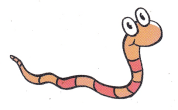(A) 8 + 8 = 16
(B) 6 + 6 = 12
(C) 9 + 9 = 18
Explanation:
7 snakes sit in the sun. 8 more snakes join them.
7 + 7 + 1 = 15
8 + 8 – 1 = 15
16 – 1 = 15
15 snakes are there now

Question 6.
Multi-Step Keisha has 4 nickels. Chen has the same number of nickels and one dime. Which addition sentence shows how many coins Keisha and Chen have?(A) 4 + 4 = 8
(B) 5 + 5 = 10
(C) 4 + 5 = 9
Explanation:
Keisha has 4 nickels. Chen has the same number of nickels and one dime.
4 + 4 + 1 = 9
4 + 5 = 9
is the addition sentence shows the coins Keisha and Chen have

Question 7.
Which doubles fact helps you solve 7 + 8 = 15?
(A) 7 + 7 = 14
(B) 9 + 9 = 18
(C) 6 + 6 = 12